• 梯度下降方法
千次阅读
2020-12-23 10:40:46

梯度下降方法(三种不同地梯度下降方法简介)

标签：#优化##机器学习##梯度下降##深度学习#

时间：2018/10/17 14:20:47

作者：小木

梯度下降是一种迭代优化的方法，它的目标是求解一个函数的最小值。我们以一个简单的例子来说明。

![](http://www.datalearner.com/resources/blog_images/4454250a-98b8-4c35-a469-7b75bca8d099.png)

例如，如上图所示，这是一个二次函数的图像：

math

y = 8(x-1.5)^2 + 1



其最低点是$(1.5,1)$。我们希望找到这个最低点。其实，对于这个简单的二次函数，我们只要求y对x的导数，并令其结果为0即可求出最低点。为了说明梯度下降的原理，我们不采用这种方式。

[TOC]

梯度下降的原理就是初始随机选择一个函数上的点，然后沿着某个方向运行选择下一个函数上的点，使得下一个点的函数值比上一个点的函数值小，这样不停迭代直到找到最小值位置。那么，这里有可能有三个问题需要注意

- 首先，为什么不使用刚才说的求导方式找最小值？这是因为并不是所有的函数都容易求导，例如对于多元函数来说，就很难，但是梯度下降的原理则依然有效。

- 其次，什么样的函数可以使用梯度下降求解？需要这个函数是凸函数，这样我们才能找到全局最小值，否则就无法确保找到最小值，因为其结果可能是极值。

- 最后，沿着什么方向寻找下一个点才是正确的，且速度最快？这就是梯度下降的核心概念：沿着梯度的方向才是最正确的方向。

如下图所示，我们随机选了一个函数图像上的点(initial point)，假设为(2.5,9)。这个时候，寻找下一个点的方向就有两个了，一个是向上(up)，另一个是向下(down)。显然，我们应该往下方走才是正确的方向。

![](http://www.datalearner.com/resources/blog_images/b3b7de85-882d-4e4e-95a9-832533b2af4d.png)

梯度下降的原理其实就是告诉我们让凸函数上的任意一点沿着梯度的方向向下就可以找到最低点了(注意，有的人说我要找的是最大值，那你就把函数加个负号，这样图形就转换过来变成找最小值了，这种简单问题不说了)。

> “A gradient measures how much the output of a function changes if you change the inputs a little bit.” — Lex Fridman (MIT)

也就是说它只是根据误差的变化来衡量所有权重的变化。 简单点说就是斜率，函数图像最陡峭的地方，也就是函数对某个权重(x)求偏导之后的方向。还是以上图为例，在最低点的左侧，函数的斜率是负值，那么左侧的横坐标的点减去梯度，它将往右侧移动，那么y的值是下降的。在右侧正好反过来了，其梯度是正值，那么最低点右侧的点减去梯度会使得这个点往左移动，这样也使得y值下降。因此，梯度就是斜率，那点的坐标轴的值减去这个梯度就能使得函数值下降，进而帮助我们寻找最小值。

刚才说的是坐标点减去梯度的方向一定是往最小值移动的，不仅如此，梯度还有个最陡峭的概念，函数上的点往任何一个方向移动都不如向梯度方向移动的时候其值下降得快。以下图为例：

![](https://encrypted-tbn0.gstatic.com/images?q=tbn:ANd9GcSLpi-wqqtuRpG_omWF_tRrRykHHa-vssml7GqpL5PpqQsAJnEe)

中间红色的就是梯度的方向，那么下山最快，其他发现虽然也可以下山，但是多走了很多弯路。在我们的上面的函数中，就是下图红色虚线的方向是梯度了：

![](http://www.datalearner.com/resources/blog_images/4a197535-e11c-4ae8-be49-7b954a823345.png)

#### 三、数学化描述

下面我们来用数学语言描述一下梯度下降。假设我们有一个凸函数：

math

y = f(x)



其形式比较复杂无法直接求出最小值，我们来使用梯度下降的方法求其最小值。那么，首先我们要计算梯度：

math

\nabla f(x) = \frac{dy}{dx}



使用梯度下降的时候，第一步要随机选择一个初始点，如$(x\_0,y\_0)$，然后，利用沿着梯度的方向搜索寻找下一个点：

math

x_1 = x_0 - \alpha \nabla f(x_0)



math

y_1 = f(x_1)



这样我们就得到了下一个点，这时候我们计算一下$y\_1-y\_0$，如果结果很小，那么说明我们已经接近底部，即最小值了，通常我们只要这个近似最小值就可以了。

在机器学习中，我们有很多求最小值的问题，因为我们有很多机器学习的任务都有一个目标函数，需要求目标函数的最小值已达到最好的拟合效果。以回归分析为例，一般的线性回归分析的方程如下：

math

\hat{y} = \sum_{j=0}^n w_jx_j



一般情况下，我们有m个训练集，我们的目标函数是一个残差平方和：

math

\begin{aligned}

J(\bold{w}) &= \frac{1}{2m} \sum_{i=1}^m (\hat{y_i} - y_i)^2 \\

& \\

&= \frac{1}{2m} \sum_{i=1}^m (\sum_{j=0}^n w_jx_{ij} - y_i)^2 \\

\end{aligned}



我们希望求出这个目标函数的最小值，这是无法直接求导得出结论的，因此我们可以使用梯度下降来求解，这个函数的梯度为：

math

\nabla = \frac{\partial J}{\partial w_j} = \frac{1}{m} \sum_{i=1}^m (\sum_{j=0}^n w_jx_{ij} - y_i) x_{ij}



#### 四、三种不同的梯度下降方法

##### 4.1、批量梯度下降(BGD)

批量梯度下降(也称为原始梯度下降)计算训练数据集中每个样本的误差，但是每次更新参数都会使用所有的训练样本：

math

w_j' = w_j - \frac{1}{m} \sum_{i=1}^m (\sum_{j=0}^n w_jx_{ij} - y_i) x_{ij}



它的优点是它具有计算效率，它可以产生稳定的误差梯度和稳定的收敛。 批量梯度下降的缺点是稳定的误差梯度有时会导致收敛状态不是模型可以达到的最佳状态。 它还要求整个训练数据集在内存中并可供算法使用。也就是说对内存的要求很高。每一次迭代下对每个参数进行更新的时候，都要计算所有的数据，因此在样本数量很大的时候比较耗时。

##### 4.2、随机梯度下降(SGD)

相反，随机梯度下降(SGD)对数据集中的每个训练样本都进行更新操作。这意味着它会逐个更新每个训练样本的参数。这可以使SGD比批量梯度下降更快，具体取决于问题。 一个优点是频繁的更新使我们有一个非常详细的改进率。

事实上，频繁更新作为批量梯度下降的方法在计算上更加昂贵。这些更新的频率也可能导致噪声梯度，这可能导致错误率跳跃，而不是缓慢下降。因此，它的下降过程是个杂乱的过程，看起来没有顺序。

以上述为例，我们写出每个样本(这里以第$i$个样本为例)的目标函数(损失函数)：

math

\frac{1}{2} (\sum_{j=0}^n w_jx_{ij} - y_i)^2



每个样本都会更新所有的参数：

math

w_j' = w_j - (\sum_{j=0}^n w_jx_{ij} - y_i) x_{ij}



随机梯度下降在每一次迭代的时候，先循环每个样本，对每个样本都更新所有的权重。

##### 4.3、小批量梯度下降(MBGD)

小批量梯度下降是首选方法，因为它结合了随机梯度下降(SGD)和批量梯度(BGD)下降的概念。 它只是将训练数据集拆分成小批量，并为每个批次执行更新。 因此，它在随机梯度下降的稳健性和批量梯度下降的效率之间创造了平衡。

常见的小批量大小介于50到256之间，但与任何其他机器学习技术一样，没有明确的规则，因为它们可能因不同的应用而异。 请注意，它是您训练神经网络时的首选算法，它是深度学习中最常见的梯度下降类型。

它的迭代首先需要将样本数据划分成很多个组，对每一次迭代来说，先循环所有的组，对每个组内的数据采用批量梯度下降的方式循环。

总结一下，随机梯度下降可能不是很快能找到最优的结果，因为它是跳跃着进行的，而批量梯度下降能快速的找到最优解，但是对内存要求较高。如下图所示是三种不同梯度下降的运行情况：

![](https://cdn-images-1.medium.com/max/1600/0*sFYJwQCCjOnXpSoD.png)

更多相关内容
• 梯度下降是迭代法的一种,可以用于求解最小二乘问题(线性和非线性都可以)。在求解机器学习算法的模型参数，即无...在机器学习中，基于基本的梯度下降法发展了两种梯度下降方法，分别为随机梯度下降法和批量梯度下降法。
• 梯度下降的三种方法： Batch gradient descent: 每一步的梯度下降都使用了所有的训练样本，所有样本梯度的平均值。缺点是计算量大，内存消耗大。优点：全局最优解；易于并行实现。 SGD 随机梯度下降，每个样本都计算...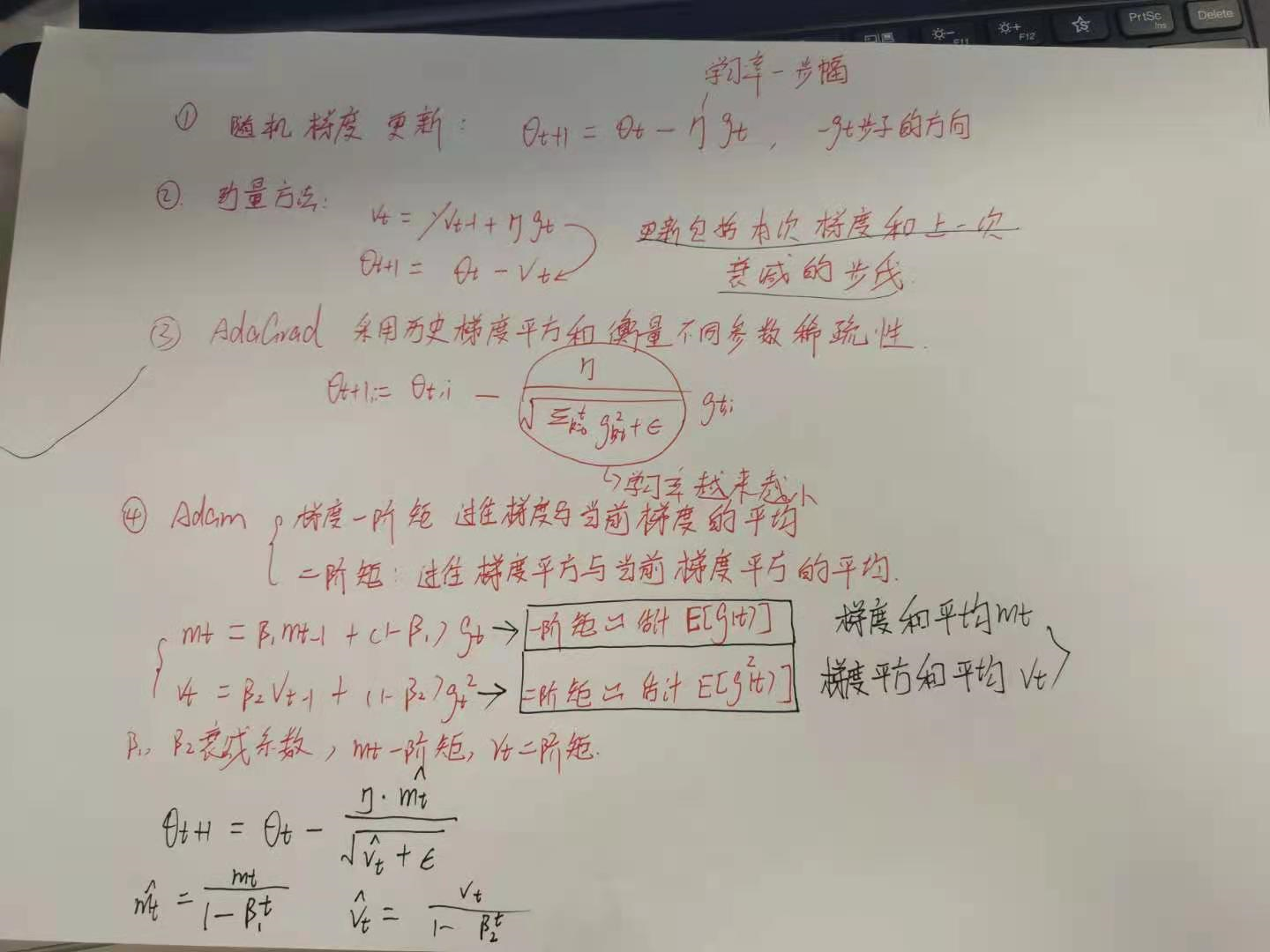首先，梯度下降最直观就是方向的选择问题，从山顶往山底，每次沿着梯度的方向(最抖的方向)往下，路程是最短的。
梯度下降的目的是最小化损失函数。

## 梯度下降的三种方法：

SGD 随机梯度下降，每个样本都计算一次梯度并下降。训练速度快，可能局部最小。

Mini-batch：综合了上述两者的优点。把数据集分成多个子集，然后每次对子集里的样本进行梯度下降。

附：样本特征的缩放以及标准化可以使得梯度下降更快！

梯度下降的推导流程和向量化例子：
假设函数如下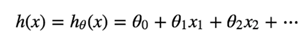损失函数是平方误差的形式：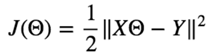要使损失函数达到最小，依次更新 delta0,delta1……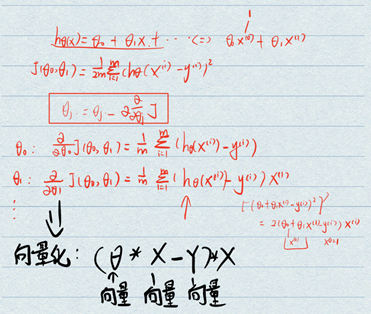展开全文最一般的就是梯度下降。

train = tf.train.GradientDescentOptimizer(learning_rate=0.1).minimize(cost)


以下是除梯度下降之外可选的方法：

tf.train.AdadeltaOptimizer
tf.train.MomentumOptimizer
tf.train.FtrlOptimizer
tf.train.RMSPropOptimizer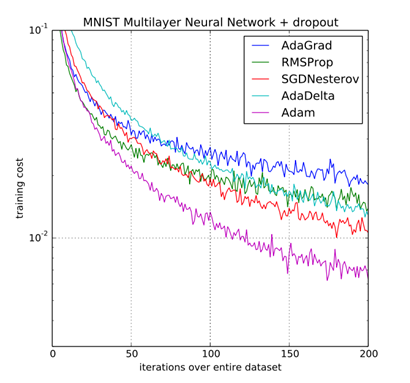Adam优化算法是首选的梯度下降方式，研究证明它可以以最快的速度逃离鞍点(局部最优点)到达全局最优点。

cost = tf.reduce_mean(tf.nn.softmax_cross_entropy_with_logits(logits=hypothesis, labels=Y))

展开全文tensorflow
• 梯度下降算法代码及详细解释，使用matlab编程

### 一、梯度下降法介绍

梯度下降法（Gradient Descent Algorithm，GD）是以负梯度方向求解目标函数 J(θ) J ( θ ) $J(\theta)$的全局最小值的一种迭代方法。

参数更新的步骤：

• 随机初始化一组参数 θ θ $\theta$
• 将目标函数 J(θ) J ( θ ) $J(\theta)$分别对每个参数 θi θ i $\theta_i$求偏导
• 每个参数的更新，是在对所有样本点都求得对该参数的偏导之后，求和取平均，作为该参数梯度更新的方向
• 每轮中对每个参数都要求偏导和更新

示例：

hθ(x)=θ0+θ1x1+θ2x2 h θ ( x ) = θ 0 + θ 1 x 1 + θ 2 x 2 $h_\theta(x)=\theta_0+\theta_1x_1+\theta_2x_2$

J(θ)=12mmi(hθ(x(i))y(i))2 J ( θ ) = 1 2 m ∑ i m ( h θ ( x ( i ) ) − y ( i ) ) 2 $J(\theta)=\frac{1}{2m} \sum_i^m(h_\theta(x^{(i)})-y^{(i)})^2$

每个样本 X X $X$是一个$1×n$$1\times n$的向量，即\$X=[x^1,x^2,x^3,…,x^n]，每个向量代表一个特征，每个参数对应一个特征的权重，所以每个样本对应的参数也是n维的。

对参数 θ1 θ 1 $\theta_1$的更新：

J(θ)θ1=1mmi(hθ(x(i))y(i))x(1) ∂ J ( θ ) ∂ θ 1 = 1 m ∑ i m ( h θ ( x ( i ) ) − y ( i ) ) ⋅ x ( 1 ) $\frac{\partial J(\theta)}{\partial _{\theta_1}}=\frac{1}{m}\sum_i^m(h_\theta(x^{(i)})-y^{(i)})\cdot x^{(1)}$

θ1=θ1η1mmi(hθ(x(i))y(i))x(1) θ 1 = θ 1 − η ⋅ 1 m ∑ i m ( h θ ( x ( i ) ) − y ( i ) ) ⋅ x ( 1 ) $\theta_1=\theta_1-\eta\cdot \frac{1}{m}\sum_i^m(h_\theta(x^{(i)})-y^{(i)})\cdot x^{(1)}$

对参数 θ2 θ 2 $\theta_2$的更新：

J(θ)θ2=1mmi(hθ(x(i))y(i))x(2) ∂ J ( θ ) ∂ θ 2 = 1 m ∑ i m ( h θ ( x ( i ) ) − y ( i ) ) ⋅ x ( 2 ) $\frac{\partial J(\theta)}{\partial _{\theta_2}}=\frac{1}{m}\sum_i^m(h_\theta(x^{(i)})-y^{(i)})\cdot x^{(2)}$

θ2=θ2η1mmi(hθ(x(i))y(i))x(2) θ 2 = θ 2 − η ⋅ 1 m ∑ i m ( h θ ( x ( i ) ) − y ( i ) ) ⋅ x ( 2 ) $\theta_2=\theta_2-\eta\cdot \frac{1}{m}\sum_i^m(h_\theta(x^{(i)})-y^{(i)})\cdot x^{(2)}$

### 二、批量梯度下降法

参数 θ θ $\theta$的每次更新，需要 计算全部数据集的样本的负梯度方向，取均值作为下一次梯度下降的方向。

特点：

• 计算量大，参数更新慢，对内存的要求很高，不能以在线的形式训练模型，也就是运行时不能加入新样本

• 理论上讲，可以得到全局最优解，参数更新比较稳定，收敛方向稳定

### 三、随机梯度下降法

每次只选择一个样本来求得偏导，进行参数 θ θ $\theta$的更新。

特点：

• 运算速度很快，同时能够在线学习

• 随机梯度下降参数更新的过程震荡很大，目标函数波动剧烈，参数更新方向有很大的波动

• 其较大的波动可能收敛到比批量梯度下降更小的局部极小值，因为会从一个极小值跳出来

目标函数下降很波动：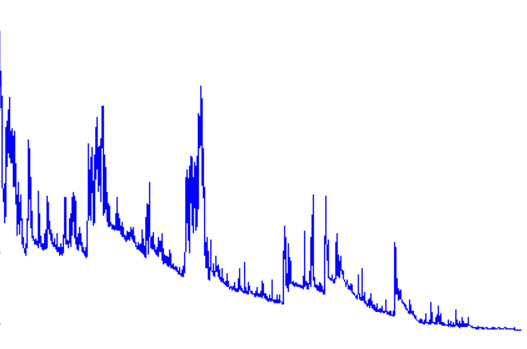梯度更新的方向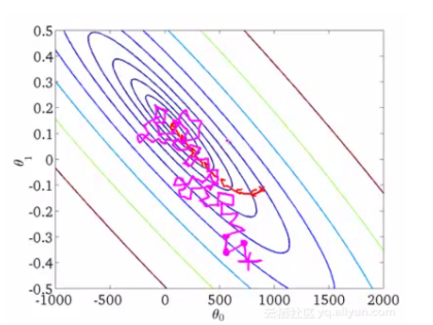示例：

hθ(x)=θ0+θ1x1+θ2x2 h θ ( x ) = θ 0 + θ 1 x 1 + θ 2 x 2 $h_\theta(x)=\theta_0+\theta_1x_1+\theta_2x_2$

J(θ)=12(hθ(x(i))y(i))2 J ( θ ) = 1 2 ( h θ ( x ( i ) ) − y ( i ) ) 2 $J(\theta)=\frac{1}{2} (h_\theta(x^{(i)})-y^{(i)})^2$

对参数 θ1 θ 1 $\theta_1$的更新：

J(θ)θ1=(hθ(x(i))y(i))x(1) ∂ J ( θ ) ∂ θ 1 = ( h θ ( x ( i ) ) − y ( i ) ) ⋅ x ( 1 ) $\frac{\partial J(\theta)}{\partial _{\theta_1}}=(h_\theta(x^{(i)})-y^{(i)})\cdot x^{(1)}$

θ1=θ1η(hθ(x(i))y(i))x(1) θ 1 = θ 1 − η ( h θ ( x ( i ) ) − y ( i ) ) ⋅ x ( 1 ) $\theta_1=\theta_1-\eta(h_\theta(x^{(i)})-y^{(i)})\cdot x^{(1)}$

### 四、小批量梯度下降法（mini-batch GD）

该方法集合了批量梯度下降和随机梯度下降两者的优势，每次的参数更新利用一小批数据来完成。

特点：

• 降低了更新参数的方差，使得收敛过程更加的稳定
• 能够利用高度优化的矩阵运算，很高效的求得每小批数据的梯度

上述方法面临的主要挑战：

• 选择合适的学习率较为困难，太小的学习率会导致收敛缓慢，太大的学习率导致波动较大，可能跳出局部最小值

• 目前可采用的方法是在训练过程中调整学习率的大小，例如模拟退火法：预定义一个迭代次数m，每次执行完m次训练便减小学习率，或者当损失函数的值低于一个阈值时，减小学习率，迭代次数和损失函数的阈值必须事先定义。

• 上述方法中，每个参数的学习率都是相同的，但这种方法是不合理的，如果训练数据是稀疏的，并且不同特征的出现频率差异较大，那么比较合理的做法是对于出现频率较低的特征设置较大的学习率，对于出现频率较大的特征设置较小的学习率。

• 近期研究表明，深层网络之所以比较难以训练，并不是因为容易进入局部最优，因为网络结构十分复杂，即使进入了局部最优也可以得到很好的效果，难以训练的原因在于学习的过程很容易落入鞍点，也就是在一个维度是极小值，另一个维度却是极大值的点，而这种情况比较容易出现在平坦区域，在这种区域中，所有方向的梯度几乎都是0，但有的方向的二阶导小于0，即出现极大值。

### 五、动量法（Momentum）

SGD（一般指小批量梯度下降）的一个缺点在于，其更新的方向完全依赖于当前batch计算出的梯度，因而十分不稳定。

Momentum算法借用了物理中的动量的概念，模拟物体运动时候的惯性，即在更新的时候在一定程度上保留之前更新的方向，同时利用当前batch的梯度对之前的梯度进行微调，这样一来，可以在一定程度上增加稳定性，从而学习的更快，并且有一定的摆脱局部最优的能力。

更新后的梯度 = 动量项 / 折损系数(gamma) x 更新前的梯度+当前的梯度

vt=γvt1+ηJ(θ)θ v t = γ v t − 1 + η ∂ J ( θ ) ∂ θ $v_t=\gamma v_{t-1}+\eta\frac{\partial J(\theta)}{\partial \theta}$

θ=θvt θ = θ − v t $\theta=\theta-v_t$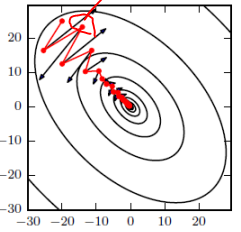上图中，红色为SGD+momentum，黑色为SGD，

注意：

• 动量项是表示在多大程度上保留原来的更新方向，值在0~1之间，是自己设置的超参数，训练开始时，由于梯度可能会很大，所以初始值一般选为0.5，当梯度慢慢减小的时候，改为0.9。

刚开始的时候，梯度方向变化很大，原始梯度起的作用较小，当前时刻的梯度方向减弱，则动量项较小，动量小则步长小

后面的时候，梯度方向变化较小了，原始梯度起的作用大，当前时刻的梯度方向增强，则动量项较大，动量大则步长大

• 学习率 η η $\eta$，当前batch的梯度以多大的程度加到之前的梯度上去

• 动量项的解释：物体运动都是有惯性的，随机梯度下降和批量梯度下降的梯度之和本时刻的梯度有关，可以立刻改变方向，但如果加上动量的话，方向不可能骤转。

• 当动量项分别设置为0.5，0.9，0.99的时候，分别表示最大速度2倍、10倍、100倍于SGD的算法

### 六、Nesterov Momentum

但当一个小球从山谷滚落的时候，盲目的沿着斜率方向前行，其效果并不令人满意，我们需要有一个更聪明的小球，能够知道往哪里前行，并知道斜率再度上升的时候减速。

在预测参数下一次的位置之前，我们已有当前的参数和动量项，先用 (θγvt1) ( θ − γ v t − 1 ) $(\theta-\gamma v_{t-1} )$作为参数下一次出现位置的预测值，虽然不准确，但是大体方向是对的，之后用我们预测到的下一时刻的值来求偏导，让优化器高效的前进并收敛。

vt=γvt1+ηJ(θγvt1)θ v t = γ v t − 1 + η ∂ J ( θ − γ v t − 1 ) ∂ θ $v_t=\gamma v_{t-1}+\eta\frac{\partial J(\theta-\gamma v_{t-1} \,\,\,\,)}{\partial \theta}$

θ=θvt θ = θ − v t $\theta=\theta-v_t$

Momentum更新方式：

计算当前的梯度值（小蓝色方向向量） $\implies$在更新后的累积方向上前进一大步

Nesterov Momentum更新方式：

按照原来的更新方向更新一步（棕色线）

$\implies$然后在该位置计算梯度值（红色线）

$\implies$利用该梯度值修正最终的更新方向（绿色线）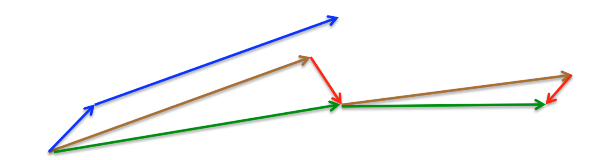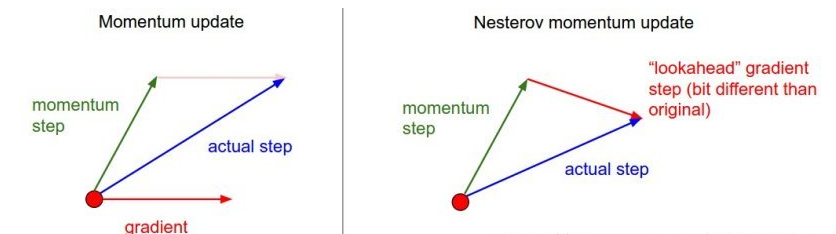该方法是基于梯度的优化方法，其主要功能是：对于不同的参数使用不同的学习率，适合于处理稀疏数据。

• 对低频出现的参数进行大的更新

• 对高频出现的参数进行小的更新

对迭代次数为 t t $t$时，对参数${\theta }_{i}$$\theta_i$求目标函数的梯度： gt,i=J(θt,i)θt,i g t , i = ∂ J ( θ t , i ) ∂ θ t , i $g_{t,i}=\frac{\partial J(\theta_{t,i}\,\,)}{\partial \theta_{t,i}}$

Adagrad将学习率 η η $\eta$进行了修正，对迭代次数t，基于每个参数之前计算的梯度值，将每个参数的学习率 η η $\eta$按如下方式修正：

θt+1,i=θt,iηGt,ii+ξgi,t θ t + 1 , i = θ t , i − η G t , i i + ξ ⋅ g i , t

其中， GtRd×d G t ∈ R d × d $G_ t ∈R^{ d×d}$ 为对角矩阵，每个对角线位置 i,i i , i $i,i$为对应参数 θi θ i $θ_ i$ 从第1轮到第t轮梯度的平方和。 ϵ ϵ $ϵ$是平滑项，用于避免分母为0，一般取值1e−8。

特点：

• 对于每个参数而言，随着其更新的总距离增多，其学习速率也随之变慢。

存在的问题：

• 学习率是单调递减的，后期的学习率非常小

• 需要手工设置一个全局的初始学习率

• 更新时，左右两边的单位不统一

此处的梯度累积和定义为：关于过去梯度值的衰减均值，当前时间的梯度均值是基于过去梯度的均值好当前梯度值平方的加权平均，$\gamma$$\gamma$是类似于动量项的权重。

E[g2]t=γE[g2]t1+(1γ)g2t E [ g 2 ] t = γ E [ g 2 ] t − 1 + ( 1 − γ ) g t 2

Δθt=ηGt+ξgt Δ θ t = − η G t + ξ ⋅ g t

Δθt=ηE[g2]t+ξgt Δ θ t = − η E [ g 2 ] t + ξ ⋅ g t

### 九、RMSprop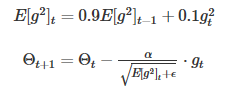• 指数衰减的过去梯度的平方均值（一阶矩）

mt=β1mt1+(1β1)gt m t = β 1 m t − 1 + ( 1 − β 1 ) g t $m_t=\beta_1m_{t-1}+(1-\beta_1)g_t$

• 也存储了指数衰减的过去梯度的均值（二阶矩）

vt=β2vt1+(1β2)g2t v t = β 2 v t − 1 + ( 1 − β 2 ) g t 2 $v_t=\beta_2v_{t-1}+(1-\beta_2)g_t^2$

使用偏差纠正系数，来修正一阶矩和二阶矩的偏差，可以近似为对期望的无偏估计：

m^t=mt1β1t m ^ t = m t 1 − β 1 t $\hat m_t=\frac{m_t}{1-\beta_1\,^t}$

v^t=vt1β2t v ^ t = v t 1 − β 2 t $\hat v_t=\frac{v_t}{1-\beta_2\,^t}$

参数的更新：

θt+1=θtηv^t+ξm^t θ t + 1 = θ t − η v ^ t + ξ m ^ t

算法的提出者建议β 1 的默认值为0.9，β 2 的默认值为.999，ϵ默认为10 −8 。

特点：经过偏置校正后，每一次迭代学习率都有个确定范围，使得参数比较平稳。

均方根传播（RMSProp）基于权重梯度最近量级的均值为每一个参数适应性地保留学习率。这意味着算法在非稳态和在线问题上有很有优秀的性能。

移动均值的初始值和 beta1、beta2 值接近于 1（推荐值），因此矩估计的偏差接近于 0。该偏差通过首先计算带偏差的估计而后计算偏差修正后的估计而得到提升。

### 十一、如何选择优化器

1、如果你的输入数据较为稀疏（sparse），那么使用适应性学习率类型的算法会有助于你得到好的结果。

展开全文• 数据挖掘-介绍二阶梯度下降方法 二阶梯度下降方法简介    在之前的文章中介绍各类的一阶梯度下降方法，同时还介绍了各种优化或者加速的方法，可以帮助更快更好地收敛到最优点。但从本质上来说，那些...算法 机器学习 深度学习 数据挖掘 神经网络
• 在求解机器学习算法的模型参数，即无约束优化问题时，梯度下降法是最常采用的方法之一。 多元函数的图像显示 方程为z=x1 ^2 + 2 * x2 ^2 – 4 * x1- 2 * x1 * x2 import numpy as np import matplotlib.pyplot as ...
• ## 梯度下降方法

万次阅读 2014-03-05 14:47:26
这几天在看《统计学习方法》这本书，发现 梯度下降法 在 感知机 等机器学习算法中有很重要的应用，所以就特别查了些资料。     一.介绍  梯度下降法（gradient descent）是求解无约束最优化问题的一种常用...
• 2.几种梯度下降方法（针对线性回归算法） 2.1 批量梯度下降法 1.批量梯度下降法的特点及原理 运算量大：批量梯度下降法中的每一项计算：，要计算所有样本（共 m 个）； 批量梯度下降法的梯度是损失函数减小最快的...算法 机器学习 深度学习
• 本文实例为大家分享了python批量梯度下降算法的具体代码，供大家参考，具体内容如下 问题： 将拥有两个自变量的二阶函数绘制到空间坐标系中，并通过批量梯度下降算法找到并绘制其极值点 大体思路： 首先，根据题意...
• 总结梯度下降方法中步长，梯度精度和迭代次数对结果的影响机器学习 算法 python 人工智能
• ## 梯度下降法

千次阅读 2019-03-31 17:08:21
• 这是一个matlab对梯度下降的实现，模拟的是x^2+y^2最小值的取得
• fmin_adam 是来自 Kingma 和 Ba  的 Adam 优化算法（具有自适应学习率的梯度下降，每个参数单独使用 Momentum）的实现。 Adam 设计用于处理随机梯度下降问题； 即当仅使用小批量数据来估计每次迭代的梯度时，或...matlab
•人工智能 神经网络 keras 计算机视觉
• 常见的最优化方法梯度下降法、牛顿法和拟牛顿法、共轭梯度法等等，本文主要介绍牛顿法和梯度下降法原理以及使用Python编程应用问题。 二、应用领域 使用牛顿法或梯度下降法，在最优化算法所解决的问题中，有着很...
• 三种梯度下降算法的比较和几种优化算法 - 知乎 pytorch学习系列（4）：常用优化算法_ch ur h的博客-CSDN博客 一、问题的提出 大多数机器学习或者深度学习算法都涉及某种形式的优化。 优化指的是改变 以最小化或...深度学习
• 做深度学习时常用到随机梯度下降方法，但是这个方法并不是在任何情况下都是有效的，也存在一些坑，可能是随机梯度下降失效导致模型效果不好。 1 随机梯度下降为什么会失效？ 先介绍下梯度下降算法 经典的梯度下降...算法 机器学习 深度学习
• 梯度下降算法代码及详细解释（非常易懂）Matlab代码，有详细图文解释，适合小白，全面介绍算法原理和公式推导
• ## 详解梯度下降算法

万次阅读 2021-08-20 10:47:46
一、 什么是梯度下降算法 梯度下降法（Gradient descent ）是一个一阶最优化算法，通常也称为最陡下降法 ，要使用梯度下降法找到一个函数的局部极小值 ，必须向函数上当前点对应梯度（或者是近似梯度）的反方向的...python
• 梯度下降是通过迭代搜索一个函数极小值的优化算法。使用梯度下降,寻找一个函数的局部极小值的过程起始于一个随机点,并向该函数在当前点梯度(或近似梯度)的反方向移动。 在线性和对数几率回归中,梯度下降可以用于...机器学习 算法
• （1） traingd：基本梯度下降法，收敛速度比较慢。 （2） traingda：自适应学习率的梯度下降法 （3） traingdm：带有动量项的梯度下降法, 通常要比traingd 速度快。 （4） traingdx: 带有动量项的自适应学习算法, ...算法 神经网络 深度学习 机器学习...